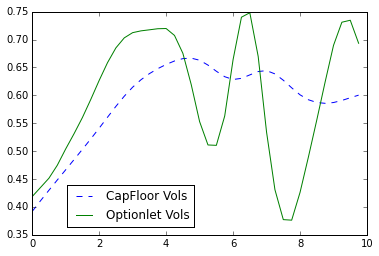A tutorial on valuing caps and floors using QuantLib Python.

Visit here for other QuantLib Python examples. If you found these posts useful, please take a minute by providing some feedback.

In this post, I will walk you through a simple example of valuing caps. I want to talk about two specific cases:

1. Value caps given a constant volatility
2. Value caps given a cap volatility surface

Caps, as you might know, can be valued as a sum of caplets. The value of each caplet is determined by the Black formula. In practice, each caplet would have a different volatility. Meaning, a caplet that is in the near term can have a different volotility profile compared to the caplet that is far away in tenor. Similarly caplet volatilities differ with the strike as well.

In :
import QuantLib as ql

In :
calc_date = ql.Date(14, 6, 2016)
ql.Settings.instance().evaluationDate = calc_date


## Constant Volatility

Let us start by constructing different componets required in valuing the caps. The components that we would need are:

1. interest rate term structure for discounting
2. interest rate term structure for the floating leg
3. construction of the cap
4. the pricing engine to value caps using the Black formula

For simplicity, we will construct only one interest rate term structure here, and assume that the discounting and the floating leg is referenced by the same. Below the term structure of interest rates is constructed from a set of zero rates.

In :
dates = [ql.Date(14,6,2016), ql.Date(14,9,2016),
ql.Date(14,12,2016), ql.Date(14,6,2017),
ql.Date(14,6,2019), ql.Date(14,6,2021),
ql.Date(15,6,2026), ql.Date(16,6,2031),
ql.Date(16,6,2036), ql.Date(14,6,2046)
]
yields = [0.000000, 0.006616, 0.007049, 0.007795,
0.009599, 0.011203, 0.015068, 0.017583,
0.018998, 0.020080]
day_count = ql.ActualActual()
calendar = ql.UnitedStates()
interpolation = ql.Linear()
compounding = ql.Compounded
compounding_frequency = ql.Annual

term_structure = ql.ZeroCurve(dates, yields, day_count, calendar,
interpolation, compounding, compounding_frequency)
ts_handle = ql.YieldTermStructureHandle(term_structure)


As a next step, lets construct the cap itself. In order to do that, we start by constructing the Schedule object to project the cashflow dates.

In :
start_date = ql.Date(14, 6, 2016)
end_date = ql.Date(14, 6 , 2026)
period = ql.Period(3, ql.Months)
calendar = ql.UnitedStates()
buss_convention = ql.ModifiedFollowing
rule = ql.DateGeneration.Forward
end_of_month = False

schedule = ql.Schedule(start_date, end_date, period,
calendar, buss_convention, buss_convention,
rule, end_of_month)


Now that we have the schedule, we construct the USDLibor index. Below, you can see that I use addFixing method to provide a fixing date for June 10, 2016. According the schedule constructed, the start date of the cap is June 14, 2016, and there is a 2 bussiness day settlement lag (meaning June 10 reference date) embedded in the USDLibor definition. So in order to set the rate for the accrual period, the rate is obtained from the fixing data provided. For all future dates, the libor rates are automatically inferred using the forward rates provided by the given interest rate term structure.

In :
ibor_index = ql.USDLibor(ql.Period(3, ql.Months), ts_handle)

ibor_leg = ql.IborLeg(, schedule, ibor_index)


Now that we have all the required pieces, the Cap can be constructed by passing the ibor_leg and the strike information. Constructing a floot is done through the Floor class. The BlackCapFloorEngine can be used to price the cap with constant volatility as shown below.

In :
strike = 0.02
cap = ql.Cap(ibor_leg, [strike])

vols = ql.QuoteHandle(ql.SimpleQuote(0.547295))
engine = ql.BlackCapFloorEngine(ts_handle, vols)

cap.setPricingEngine(engine)
print cap.NPV()

54369.8580629


## Using Volatility Surfaces

In the above exercise, we used a constant volatility value. In practice, one needs to strip the market quoted capfloor volatilities to infer the volatility of each and every caplet. QuantLib provides excellent tools in order to do that. Let us assume the following dummy data represents the volatility surface quoted by the market. I have the various strikes, expiries, and the volatility quotes in percentage format. I take the raw data and create a Matrix in order to construct the volatility surface.

In :
strikes = [0.01,0.015, 0.02]
expiries = [ql.Period(i, ql.Years) for i in range(1,11)] + [ql.Period(12, ql.Years)]
vols = ql.Matrix(len(expiries), len(strikes))
data = [[47.27, 55.47, 64.07, 70.14, 72.13, 69.41, 72.15, 67.28, 66.08, 68.64, 65.83],
[46.65,54.15,61.47,65.53,66.28,62.83,64.42,60.05,58.71,60.35,55.91],
[46.6,52.65,59.32,62.05,62.0,58.09,59.03,55.0,53.59,54.74,49.54]
]

for i in range(vols.rows()):
for j in range(vols.columns()):
vols[i][j] = data[j][i]/100.0


The CapFloorTermVolSurface offers a way to store the capfloor volatilities. These are however CapFloor volatilities, and not the volatilities of the individual options.

In :
calendar = ql.UnitedStates()
bdc = ql.ModifiedFollowing
daycount = ql.Actual365Fixed()
settlement_days = 2
capfloor_vol = ql.CapFloorTermVolSurface(settlement_days, calendar, bdc, expiries, strikes, vols, daycount)


The OptionletStripper1 class lets you to strip the individual caplet/floorlet volatilities from the capfloor volatilities. We have to 'jump' some hoops here to make it useful for pricing. The OptionletStripper1 class does not allow you to be consumed directly by a pricing engine. The StrippedOptionletAdapter takes the stripped optionlet volatilities, and creates a term structure of optionlet volatilities. We then wrap that into a handle using OptionletVolatilityStructureHandle.

In :
optionlet_surf = ql.OptionletStripper1(capfloor_vol, ibor_index)
ovs_handle = ql.OptionletVolatilityStructureHandle(
)


Below, we visulaize the capfloor volatility surface, and the optionlet volatility suface for a fixed strike.

In :
import matplotlib.pyplot as plt
import numpy as np
%matplotlib inline

In :
tenors = np.arange(0,10,0.25)
strike = 0.015
capfloor_vols = [capfloor_vol.volatility(t, strike) for t in tenors]
opionlet_vols = [ovs_handle.volatility(t, strike) for t in tenors]

plt.plot(tenors, capfloor_vols, "--", label="CapFloor Vols")
plt.plot(tenors, opionlet_vols,"-", label="Optionlet Vols")
plt.legend(bbox_to_anchor=(0.5, 0.25))

Out:
<matplotlib.legend.Legend at 0x894efd0>The BlackCapFloorEngine can accept the optionlet volatility surface in order to price the caps or floors.

In :
engine2 = ql.BlackCapFloorEngine(ts_handle, ovs_handle)
cap.setPricingEngine(engine2)
print cap.NPV()

54384.928315


The QuantLib C++ class allow for one to view the projected cashflows in terms of individual caplets. I just realized that the python extension does not have this feature added to it. Will give a PR one of these days and update this post.

Hope you find this useful.

quantlib   python   finance

Related PostI am Goutham Balaraman, and I explore topics in quantitative finance, programming, and data science. You can follow me @gsbalaraman.

Checkout my bookUpdated posts from this blog and transcripts of Luigi's screencasts on YouTube is compiled into QuantLib Python Cookbook .